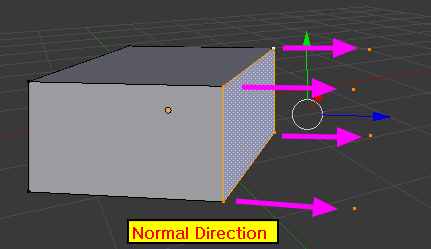# I want to move a vertices to Normal directon of origin vertex by Python.

I am a person who want to learn a python.

At this time, I want to move a vertex to normal direction of origin vertex through python.

Lock at below image.how to move or copy a vertex to normal direction ?

Thank you.

### AttachmentsIn your first picture, it doesn’t look like using vertex normals, but rather interpolated face normals (turn on vertex normal in N panel and see yourself, they are “point” apart from each other, so not parallel like in your picture).

In your second picture, it looks more like the face normal?

(Face normals in light blue, vertex normals in dark blue)

But first image is it’s true normal direction.
Show to you a below image.``````import bpy
import bmesh
from bpy.props import FloatProperty
from mathutils import *

class SimpleOperator(bpy.types.Operator):
"""Tooltip"""
bl_idname = "mesh.move_verts_normal"
bl_label = "Move Vertices along Normal"
bl_options = {'REGISTER', 'UNDO'}

factor = FloatProperty(
name="Factor",
min=-1000.0,
max=1000.0,
soft_min=-10.0,
soft_max=10.0
)

@classmethod
def poll(cls, context):
return (context.object is not None and
context.object.type == 'MESH' and
context.object.data.is_editmode)

def execute(self, context):

ob = bpy.context.object
me = ob.data
bm = bmesh.from_edit_mesh(me)

for v in bm.verts:
if v.select:
v.co += v.normal * self.factor

bmesh.update_edit_mesh(me, True, False)
return {'FINISHED'}

def register():
bpy.utils.register_class(SimpleOperator)

def unregister():
bpy.utils.unregister_class(SimpleOperator)

if __name__ == "__main__":
register()
``````

Run the script, move cursor over 3D View, hit spacebar and search for Move Vertices along Normal. Hit enter and see redo panel to tweak factor.

1 Like